New update is available. Click here to update.

# Greedy Algorithm in Graph Theory

## Introduction To Greedy Algorithms

A greedy algorithm is an algorithmic paradigm that builds up a solution piece by piece, always choosing the next piece that offers the most immediate benefit. This means that the choices made are only locally optimal, in the hope that the solution will be optimal globally.

We use greedy algorithms when we have an objective function that needs to be either minimized or maximized.

Unlike Backtracking, greedy algorithms have to come up with the most optimal choice in one shot. It cannot go back and change its decision.

Did you know, Greedy Algorithms were the asked in Google Kickstart 2020-a CodeJam Competition.

## Real-life example for Greedy Algorithms:

Consider a boy named Ram. He has a box which can accommodate at most 3 chocolates. His friend offers him 4 chocolates namely A, B, C and D of Rs.10, Rs.20, Rs.30 and Rs.40 respectively. Ram can only choose as many chocolates as the box can accommodate. This means Ram can choose 3 chocolates at most.

Which 3 chocolates should he choose so that his profit is maximized??? Also, he can make only 3 choices. In each choice, he can pick one chocolate. Yes, you got it right… He should choose B, C, and D.

Let’s try to understand this situation algorithmically by applying the greedy approach.  Initially, Ram’s box is empty and his friend has four chocolates.

Step 1: According to the definition of a greedy algorithm, Ram will choose the chocolate that will offer him the most immediate and largest profit. In this case, Ram will choose D because he will get a profit of Rs.40 which is greater than the profit made by choosing any other chocolate.

Step 2: Now Ram’s box has the capacity to accommodate 2 more chocolates. Ram has to choose 2 chocolates out of 3 such that “immediate” profit is maximised. He will choose C because of the same reason stated in step1.

Step 3: Now Ram’s box has the capacity to accommodate only 1 chocolate. Ram has to choose 1 chocolate out of 2 such that “immediate” profit is maximised. He will choose B because of the same reason stated in step1.

Now Ram’s box is full and profit is also maximised. You can try various combinations of choosing 3 chocolates out of the four chocolates in a given scenario and you will find that the profit is maximum for the above-mentioned combination with the least number of steps. So, In this case, our objective function was the profit that had to be maximised.

Some of the standard problems that can be solved using the greedy algorithm include the famous fractional knapsack problem, job sequencing problem, etc. Here, we will look at various graph greedy algorithms that are greedy algorithms and data structure

## Greedy Algorithms in Graphs

### Spanning Tree and Minimum Spanning Tree

In terms of graph theory, a spanning tree T of an undirected graph G is a tree which includes all of the nodes of the graph G. The tree T is also a subgraph of the given graph G. A single graph can have more than one spanning trees.

A minimum spanning tree (MST) for a graph is a spanning tree with weight less than or equal to the weight of every other spanning tree. The weight of a spanning tree is the sum of weights given to each edge of the spanning tree.

If a graph G has V number of vertices, its minimum spanning tree will have (V-1) number of edges. The two famous algorithms for finding the minimum spanning tree for a given graph.

They are Prim’s algorithm and Kruskal’s algorithm. Both of these are discussed in the following sections.

## Kruskal’s Algorithm

Kruskal’s algorithm is used to find the minimum spanning tree for a given graph G.

The algorithm is as follows-

• Sort the edges of the graph in a non-decreasing order with respect to their weights
• Pick the edge with the smallest weight. Check if the edge forms a cycle with the MST constructed so far. If it forms a cycle, discard it, else include it in the MST.
• Repeat step-2 till there is (V-1) number of edges in the graph (and all vertices are covered). Here, V represents the number of vertices in graph G.

Let’s understand the working of the above algorithm with an example.

Consider the graph: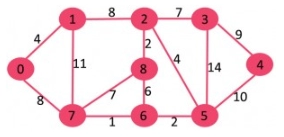Kruskal’s Algorithm

It has nine vertices and 14 edges. So, its MST will have (9-1) i.e eight edges. After sorting the edges according to increasing order of their weights, we get the following: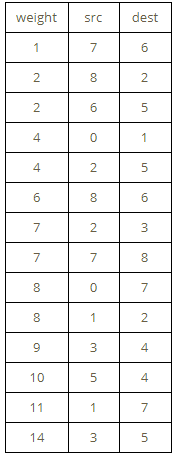Kruskal’s Algorithm Table

Here, src refers to the source vertex of a given edge, dest refers to the destination vertex of a given edge and weight refers to the weight of the given edge. Now let’s implement Kruskal’s algorithm as stated above.

Pick all edges one by one from the sorted list of edges.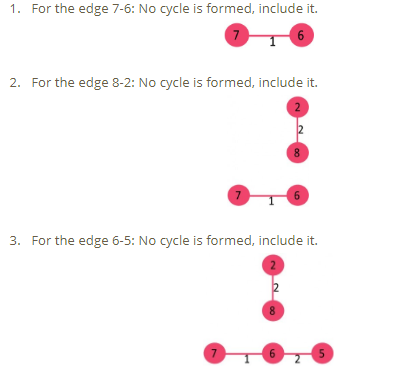Kruskal’s Algorithm-1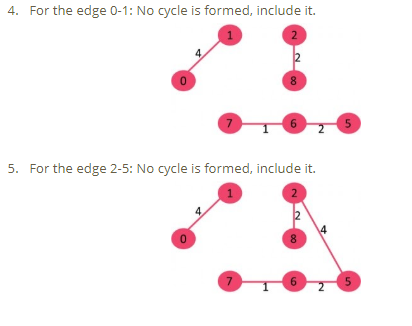Kruskal’s Algorithm-2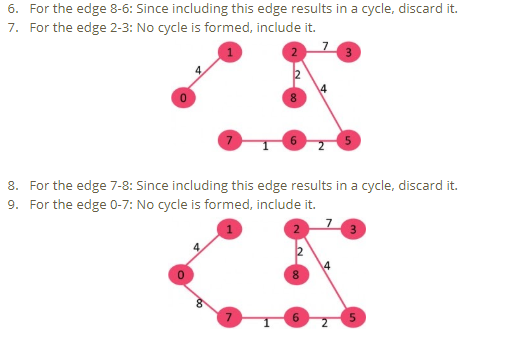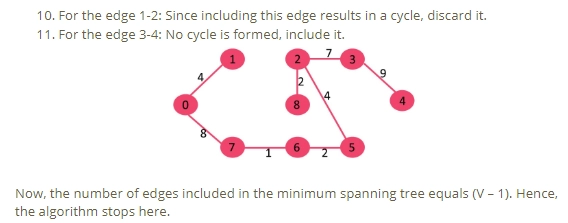Kruskal’s Algorithm-3

You can refer to Coding Ninjas courses for the best in-depth explanations for understanding these concepts.

### Time Complexity of Kruskal’s algorithm:

The time complexity for Kruskal’s algorithm is O(ElogE) or O(ElogV). Here, E and V represent the number of edges and vertices in the given graph respectively. Sorting of all the edges has the complexity O(ElogE). After sorting, we apply the find-union algorithm for each edge. The find and union operations have the worst-case time complexity is O(LogV). So overall complexity becomes O(ElogE + ElogV). The value of E can be V^2 in the worst case. Hence, O(LogV) is O(LogE) become the same. Therefore, the overall worst-case time complexity becomes O(ElogE) or O(ElogV).

## Prim’s Algorithm

Like Kruskal’s algorithm, Prim’s algorithm is also used to find the minimum spanning tree of a given graph. In Kruskal’s algorithm, we were adding an edge to an existing MST. But, Here, we will add a vertex to the existing (growing) MST.

• Maintain two disjoint sets of vertices: One set will contain the vertices that are a part of the growing spanning tree. The other set will contain the vertices that are not a part of the growing spanning tree.
• Select the cheapest vertex that is connected to the growing spanning tree. This vertex should not be there in the already growing spanning tree. Add this vertex into the growing spanning tree. This can be achieved using Priority Queues. Insert the vertices, that are connected to growing spanning tree, into the Priority Queue.
• Check for cycles: In order to check for cycles, mark the nodes which have been already selected. In the priority queue, insert only those nodes that are not marked.

In Prim’s Algorithm, we have to start with an arbitrary node and mark it. In each iteration, we will mark a new vertex that is adjacent to the one that we have already marked. Prim’s algorithm being a greedy algorithm, it will select the cheapest edge and mark the vertex.

Get a Head start on Summer with a programming checklist by Coding Ninjas 2021.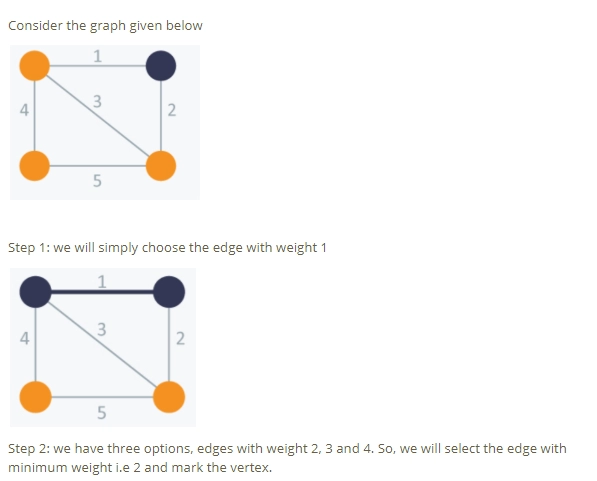Prim’s Algorithm-1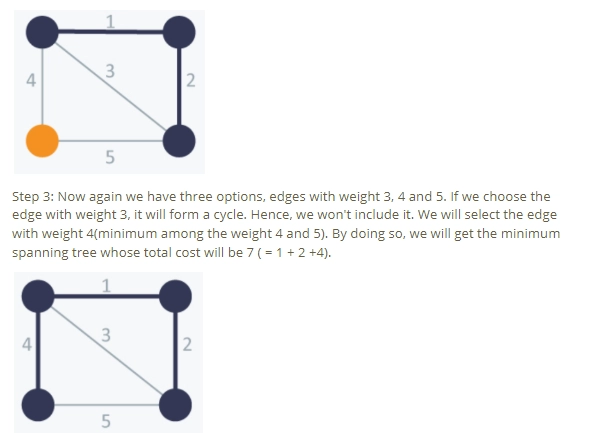Prim’s Algorithm-2

Need help with the Google Kickstart Exams, join our free trials courses to practice exam problems at Codestudio only.

### Time Complexity:

The worst case time complexity of the Prim’s Algorithm is O((V+E)logV). This is because each vertex is inserted in the priority queue only once and insertion in priority queue takes logarithmic time.

### Applications of Minimum Spanning Trees:

• Direct application in the design of networks (a network of computers, cellular networks road networks, etc.)
• Handwriting Recognition
• Cluster analysis
• Image segmentation

### Why are greedy algorithms used?

Greedy algorithm helps to arrive at the most optimal solution in the best possible time.

### List a few characteristics of Greedy algorithms.

Greedy algorithm is a simple method to solve the problem and derive an optimal solution at each step. These algorithms are fast and more intuitive compared to any other algorithms.

### Name some popular greedy algorithms.

Some popular greedy algorithms are prim's algorithm, Kruskal's algorithm, Dijkstra's algorithm, and Knapsack problem.

## Conclusion

Also, refer to our Guided Path on CodeStudio to upskill yourself in Data Structures and AlgorithmsCompetitive ProgrammingJavaScript, and many more! If you wish to test your competency in coding, you may check out the mock test series and participate in the contests hosted on CodeStudio!

But suppose you have just started your learning process and are looking for questions asked by tech giants like Amazon, Microsoft, Uber, etc. In that case, you must look at the problemsinterview experiences, and interview bundle for placement preparations.

Nevertheless, you may consider our paid courses to give your career an edge over others!

Do upvote our blogs if you find them helpful and engaging!

Happy Learning!